Published: 31 August 2016

# Anti-rollover control of a heavy-duty vehicle based on lateral load transfer rate

Shaohua Li1
Junwei Zhou2
Jianying Ren3
1, 2, 3School of Mechanical Engineering, Shijiazhuang Tiedao University, Shijiazhuang Hebei 050043, China
Corresponding Author:
Shaohua Li
Views 150

#### Abstract

With the rapid development of the highway network construction and heavy-duty vehicle market, the rollover accidents of heavy-duty vehicle continue to increase. In order to improve rollover stability of vehicle, a four degree of freedom (DOF) heavy-duty vehicle model is established. An anti-rollover control strategy is designed by using differential braking system to control the lateral load transfer rate (LTR). The dynamic simulation of vehicle with and without control is fulfilled in Matlab/Simulink. Then, the vehicle responses under typical angle step input are compared and analyzed with different road surface adhesion coefficient, vehicle speed, steering wheel angle and vehicle load. The results show that the proposed control strategy is able to improve vehicle rollover stability greatly and is also beneficial to vehicle yaw stability. The increase of road surface adhesion coefficient, vehicle speed, steering wheel angle or vehicle load has positive correlation with the rollover control effect.

## 1. Introduction

Heavy-duty vehicle has poor stability in the fast steering or turning radius too small or too large. The vehicle rollover process shows fast changing, significant nonlinear relationship, the complex coupling of tire and pavement and so on. The rollover accident is difficult to avoid if the driver correct operation is improper. Therefore, study of the vehicle anti-rollover control technology is very necessary.

Because of the ABS mature, the differential braking applications are becoming more convenient and less costly. Scholars have studied the different models of differential braking about the rollover control. Gaspar  used the lateral load transfer rate LTR as the rollover indicator, and used roll bar and differential braking integrated approach to enhance the rollover stability. Suetake  established vehicle dynamics model and proposed self-adaptive system of rollover control. Imine  developed an active steering assist system to avoid rollover of heavy vehicles. Yim  proposed linear quadratic static output feedback system and study the control of sensitivity of parameter in rollover process by CarSim. Researchers in China have done a lot of valuable research about the control of vehicle rollover [5-8]. However, the current study focused on two axes sedan or sport utility vehicles and anti-rollover control research for the three-axis heavy-duty vehicles are still rare.

In this paper, a four degree of freedom vehicle dynamics model is established for three axle heavy duty vehicles in Matlab/Simulink. In order to improve the rollover stability of vehicle, a differential braking control strategy taking the dynamic load transfer rate of LTR as control target is designed and simulation analysis is carried on different conditions.

## 2. Vehicle dynamic model

Considering the vehicle longitudinal, lateral, yaw and roll motion, this paper establishes the four degree of freedom heavy vehicle rollover model. According to D’Alembert principle, differential equations can be deduced about vehicle longitudinal, lateral, yaw and roll motion:

1
$\left\{\begin{array}{l}m\left({\stackrel{˙}{V}}_{x}-{V}_{y}{\omega }_{r}\right)=\sum _{i=1}^{6}\left[{F}_{si}\mathrm{cos}\left({\delta }_{i}\right)-{F}_{\alpha i}\mathrm{sin}\left({\delta }_{i}\right)\right],\\ m\left({\stackrel{˙}{V}}_{y}+{V}_{x}{\omega }_{r}\right)=\sum _{i=1}^{6}\left[{F}_{\alpha i}\mathrm{cos}\left({\delta }_{i}\right)+{F}_{si}\mathrm{sin}\left({\delta }_{i}\right)\right],\\ {I}_{z}{\stackrel{˙}{\omega }}_{r}=\sum _{i=1}^{6}\left[\left({F}_{\alpha i}\mathrm{c}\mathrm{o}\mathrm{s}\left({\delta }_{i}\right)+{F}_{si}\mathrm{s}\mathrm{i}\mathrm{n}\left({\delta }_{i}\right)\right){l}_{xi}-\left(-{F}_{\alpha i}\mathrm{s}\mathrm{i}\mathrm{n}\left({\delta }_{i}\right)+{F}_{si}\mathrm{c}\mathrm{o}\mathrm{s}\left({\delta }_{i}\right)\right){l}_{yi}\right],\\ {I}_{x}\stackrel{¨}{\varphi }=mgh\mathrm{s}\mathrm{i}\mathrm{n}\varphi +m\left({\stackrel{˙}{V}}_{y}+{V}_{x}{\omega }_{r}\right)h\mathrm{c}\mathrm{o}\mathrm{s}\varphi -{k}_{\varphi }\varphi -{c}_{\varphi }\stackrel{˙}{\varphi },\end{array}\right\$

where $M$, ${I}_{x}$, ${I}_{z}$ are gross vehicle mass and moment of inertia. ${V}_{x}$, ${V}_{y}$, ${\omega }_{r}$, are longitudinal, lateral speed and yaw rate. ${\delta }_{i}$ is tire steering angle ($i=$ 3-6, the rear wheel steering angle ${\delta }_{i}=$ 0). ${l}_{xi}$, ${l}_{yi}$are the distance vehicle’s center of mass to the wheel center longitudinal and lateral. $h$ is the height of the center of mass of the vehicle, ${k}_{\varphi }$ is equivalent suspension roll stiffness. ${C}_{\varphi }$ is equivalent roll damping.

Cornering force of each tire is:

2
${F}_{\alpha \mathrm{i}}={k}_{\mathrm{i}}{\alpha }_{i},\mathrm{}\mathrm{}\mathrm{}\mathrm{}\left(i=1~6\right),$

where, ${k}_{i}$ is the cornering stiffness of each tire, and slip angle of each tire can be calculated:

3
${\alpha }_{\mathrm{i}}=\left\{\begin{array}{l}\mathrm{a}\mathrm{r}\mathrm{c}\mathrm{t}\mathrm{a}\mathrm{n}\left(\frac{{v}_{y}+{l}_{1}{\omega }_{r}}{{v}_{x}}\right)-{\delta }_{i},\mathrm{}\mathrm{}\mathrm{}\mathrm{}\mathrm{}i=1,\mathrm{}2,\\ \mathrm{a}\mathrm{r}\mathrm{c}\mathrm{t}\mathrm{a}\mathrm{n}\left(\frac{{v}_{y}-{l}_{2}{\omega }_{r}}{{v}_{x}}\right),\mathrm{}\mathrm{}\mathrm{}\mathrm{}\mathrm{}\mathrm{}\mathrm{}\mathrm{}\mathrm{}\mathrm{}\mathrm{}\mathrm{}\mathrm{}i=3~6.\end{array}\right\$

Longitudinal force of tires can be calculated by the brake force distribution formula, according to differential braking control.

## 3. Control strategy based on LTR

Lateral load transfer ratio LTR of tire is the ratio of the difference of the vertical load forces of the left wheels and the right wheels of the vehicle and the vehicle vertical load force. According to vehicle dynamics equation and LTR definitions, we can deduce the following equation:

4
$LTR=-\frac{2\left(c\stackrel{˙}{\varphi }+k\varphi \right)}{mgT}.$

During the process vehicle roll from the normal driving, LTR has a positive correlation with lateral acceleration of the vehicle, and LTR can be used as indicators of rollover. When the vehicle did not roll, $LTR=$ 0; When the wheel on the right side of the vehicle off the ground, $LTR=$ 1; when the left wheel off the ground, $LTR=$ –1. When the wheels of the vehicle off the ground, rollover occurs, then the value of rollover threshold of LTR is reached.

In this paper, for three-axis heavy-duty vehicles, we use differential braking control to get compensation torque to achieve the purpose of controlling vehicle stability. If the tire is not locked, the relationship between braking force of the tire and its load is approximately proportional to the vertical. If the left or right wheel is braked, dynamic allocation of the braking force goes by the following formula :

5
${F}_{s1}=\frac{\left(1+LTR\right){l}_{2}{\varphi }_{b}}{2\left({l}_{1}+{l}_{2}\right)},{F}_{s2}=\frac{\left(1-LTR\right){l}_{2}{\varphi }_{b}}{2\left({l}_{1}+{l}_{2}\right)},{F}_{s3}=\frac{\left(1+LTR\right){l}_{1}{\varphi }_{b}}{4\left({l}_{1}+{l}_{2}\right)},$
${F}_{s4}=\frac{\left(1-LTR\right){l}_{1}{\varphi }_{b}}{4\left({l}_{1}+{l}_{2}\right)},{F}_{s5}=\frac{\left(1+LTR\right){l}_{1}{\varphi }_{b}}{4\left({l}_{1}+{l}_{2}\right)},{F}_{s6}=\frac{\left(1-LTR\right){l}_{1}{\varphi }_{b}}{4\left({l}_{1}+{l}_{2}\right)},$

where ${\phi }_{b}$ is braking force coefficient the ratio of the ground brake force to the vertical load. The numerical value is different when the slip ratio is different.

Anti-rollover controller based on Matlab/Simulink is shown in Fig. 1.

Fig. 1LTR control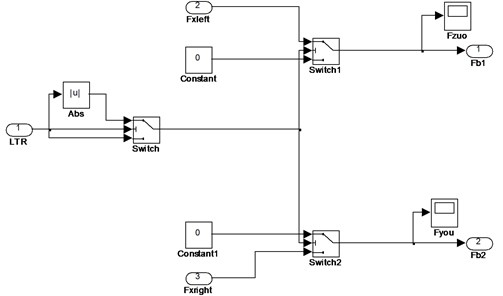Fig. 2Angle step response in basic condition (dry asphalt, steering angle is 150 deg, the speed is 80 km/h, no-load)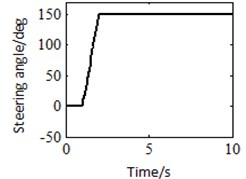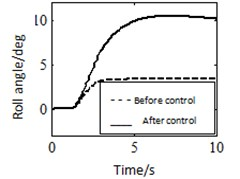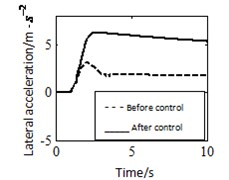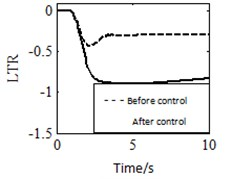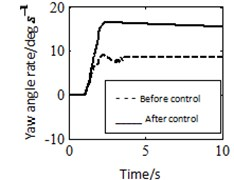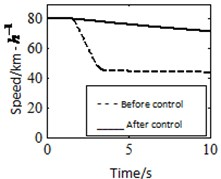## 4. Simulation of typical conditions

By using the robust control method presented for heavy duty vehicle differential braking, it takes angle step response of typical operating mode as an example, based on the three-axis heavy-duty vehicle , the different adhesion coefficient, different vehicle speed, different angle, and different load are simulated in Matlab, which is used to analysis and testify control effect. Choosing dry bituminous pavement. Setting the adhesion coefficient is 0.85, the initial speed of heavy-duty vehicle is 80 km/h, the vehicle’s mass is 10840 kg, and the steering angle is 150 deg as the basic working condition. The angle step response of vehicle is shown in Fig. 2. It can be seen from Fig. 2 that when no-load vehicle is exerted control on dry bituminous pavement, the roll angle decreased by 53 %, the lateral acceleration decreased by 60 %, the lateral load transfer ratio decreased by 57 %, the yaw angle rate decreased 30 %, the speed from 80 km/h to 45 km/h evenly in 2 s, the rollover stability is improved, and the control effect is remarkable.

### 4.1. Different road friction coefficient

Choosing pavement is ice, the adhesion coefficient is set equal to 0.1, other parameters would be same with the basic operating condition, the response of vehicle which has a step input is shown in Fig. 3. It can be seen from Fig. 3 that when the vehicle is exerted control on the ice, the roll angle decreased by 30 %, the lateral acceleration decreased by 18 %, the lateral load transfer ratio decreased by 42 %, the yaw angle rate decreased 20 %, the speed from 80 km/h to 50 km/h evenly in 8 s. By comparing Fig. 3 and Fig. 2, when the vehicle is exerted control, the value of roll angle, lateral acceleration, LTR and yaw angle rate are bigger in low attachment surface, than in high attachment surface. The response can’t be controlled into a steady value, there is a degradation in control performance, but the control effect is still remarkable. Therefore, the anti-rollover control effect is positively correlated with the adhesion coefficient.

Fig. 3Angle step response in low attachment surface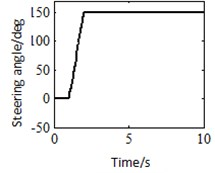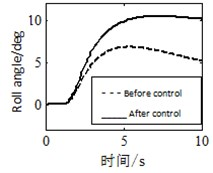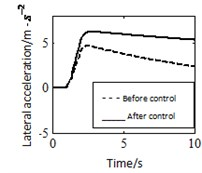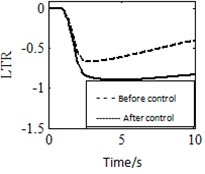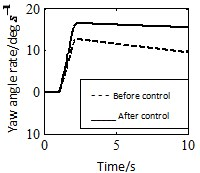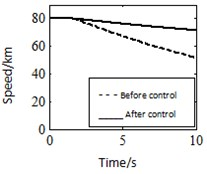Fig. 4Angle step response in low speed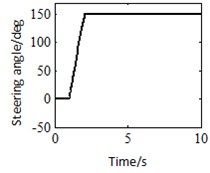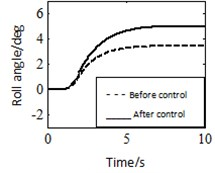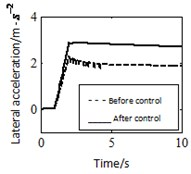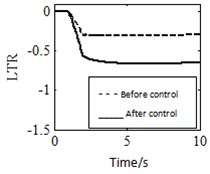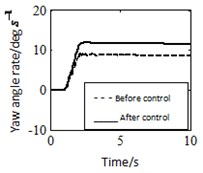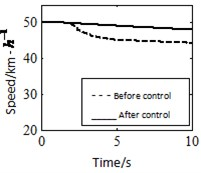### 4.2. Different initial speed

Setting the initial speed of vehicle to 50 km/h, other parameters would be same with the basic operating condition, the angle step response of vehicle is shown in Fig. 4. It can be seen from Fig. 4 that when setting the initial speed of vehicle is 50 km/h, then the vehicle is exerted control, the roll angle decreased by 18 %, the lateral acceleration decreased by 15 %, the lateral load transfer ratio decreased by 14 %, the yaw angle rate decreased 8 %, the speed from 80 km/h to 44 km/h evenly in 2 s. By comparing Fig. 4 and Fig. 2, roll angle, lateral acceleration, load transfer ratio and yaw angle rate decrease when the steering angle increases at very low vehicle speed. The anti-rollover control can enhance rollover stability whether vehicle speed is high or low. High speed is better than low speed for the anti-rollover control effect. The anti-rollover control effect is positively correlated with the vehicle speed. Hence, roll angle, lateral acceleration, load transfer ratio and yaw angle rate increase when the steering angle increases and has been very effective for anti-rollover control.

In addition, the effects of steering angle and vehicle load are also researched. Due to article length limit, only some conclusions are listed here. It has been found that the anti-rollover effect is positively correlated with the steering angle’s value and the roll stability becomes poor when vehicle load increases.

## 5. Conclusions

In this paper a four degree of freedom (DOF) heavy-duty vehicle model is established for the three-axis heavy-duty vehicle. The anti-rollover control strategy is designed by using differential braking system of the lateral load transfer rate (LTR). The anti-rollover control system is constructed in Matlab/Simulink, and simulates the angle step response under different road surface adhesion coefficient, initial speed of vehicle, steering angle, and vehicle load. The study found that when increase of road surface adhesion coefficient, vehicle speed, steering angle and vehicle’s load, the anti-rollover control strategy has a significantly effect. In all kind of driving conditions, the anti-rollover control can be effective prevent vehicle rollover, and significantly improved vehicle yaw stability. Thus, the differential braking anti-rollover control has a wide scope, and a promising prospect.

21 July 2016
Accepted
26 July 2016
Published
31 August 2016
SUBJECTS
Vibration in transportation engineering
Keywords
heavy-duty vehicle
anti-rollover control
LTR
differential-braking